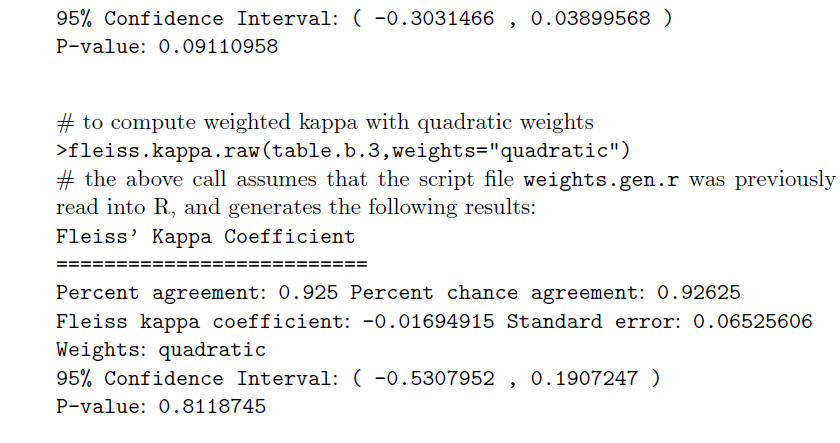Handbook of Inter-Rater Reliability, 4th Edition
Kilem L. Gwet. Ph.D.

Errors found in the fourth edition of the book

 Page 36 (Last Paragraph) The last paragraph on this page should read as follows: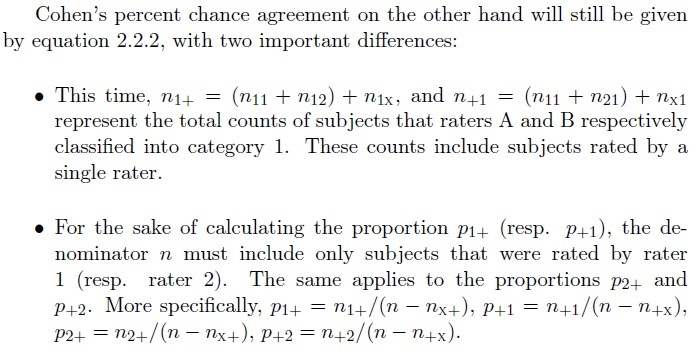Page 37 (Example 2.1) - Many thanks to D. Klein. The calculation ofis wrong. The last paragraph of Example 2.1 should read as follows:The last sentence of paragraph following example 2.1 is revised as follows:Page 44 (First paragraph of Example 2.2) The last sentence of the first paragraph of Example 2.2 (just before Table 2.8) should end as follows: " .... to facilitate the use of equations 2.3.1 through 2.3.5. In the last bullet point on that same page, "(equation 2.6)" should be replaced with "(equation 2.3.2)" Page 46 (First paragraph) The frist paragraph on page 46, including equation 2.3.7 but excluding Table 2.9 must be replaced with the following:Page 47 (First 3 bullet points) The first 3 bullet point on this page must be replaced with the following: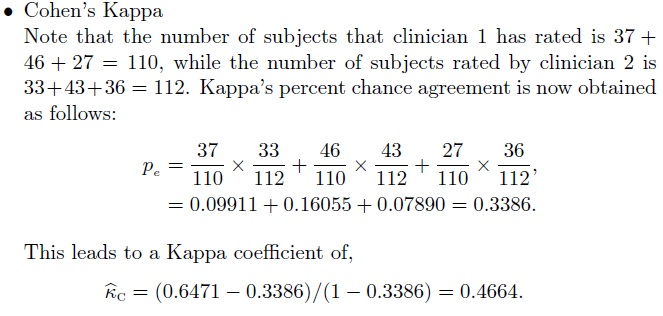Page 48 (Table 2.11) Table 2.11 of page 48 must be replaced with the following table:Page 54 (Equation 2.4.6) - Many thanks to Nilden Tutalar. Equation 2.4.6 is erroneous (the denominator in the expression of pe is (q-1), and not q*(q-1) as specified in the book). The correct equation is the following: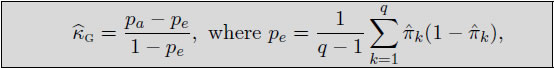Page 54 (Equations 2.4.8 and 2.4.9) Equation 2.4.8 is inaccurate. It must be replaced by the following equation:and equation 2.4.9 would be replaced with the following: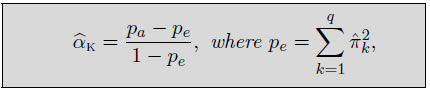Page 55 (Equations 2.4.10) Equation 2.4.10 contains a typo, and must read as follows: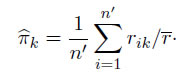Page 56 (Last bullet point) The last bullet point on page 56 must be replaced with the following paragraph: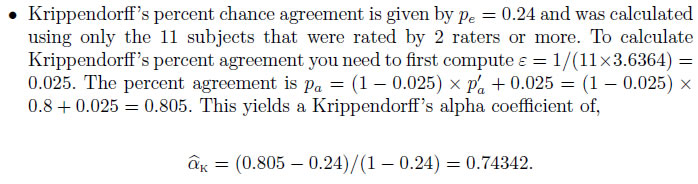Page 64 (Tables 2.24 and 2.25) - Many thanks to Bruce Burkemper (South Carolina) Below are the corrected tables, where the revisions are circled in red.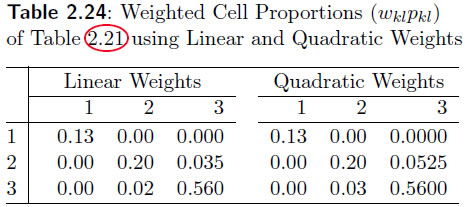Page 81 (first paragraph) Replace "... these quadratic weights from equation 3.5 ..." with "... these quadratic weights from equation 3.2.5 ..." Page 84 (Example 3.2) The second bullet point of Example 3.2 should read: Gwet's $\mbox{AC}_2$ is, $\widehat{\kappa}_{_{G}}=(0.9375-0.6405)/(1-0.6405) = 0.8261.$ The third bullet point of Example 3.2 should read: Scott's Pi is, $\widehat{\kappa}_{_{S}}=(0.9375-0.7314)/(1-0.7314) = 0.7673.$ The second row of Table 3.8 should read: $\mbox{Gwet's } \mbox{AC}_1/\mbox{AC}_2$ | $0.6322$ | $0.8261$. The third row of Table 3.8 should read: $\mbox{Scott's Pi}$ | $0.6097$ | $0.7673$. Page 117 (Second paragraph) The first sentence of the second paragraph should read as follows: One way to verify how well equation (4.4.6) works is to simulate a population of subjects similar to what is described in Table 4.3, to define the "true" inter-rater reliability according to equation 4.4.1, and to study the statistical properties of the coefficient given by equation 4.2.1. Page 118 (Second paragraph) (the hat indicating that it is an approximation of the fixed and unknown abstractof equation 4.4.1). ..... Page 118 (Second paragraph) . . . a good approximation of the population parameterof equation 4.4.1 as shown by Gwet (2008a). Page 124 (Table 4.8) Gwet'sshould be replaced by Gwet's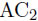Page 137(first paragraph) . . . the population-based coefficient takes (e.g. equation 5.2.3) ... Page 148 (last sentence of the first paragraph) "... is defined by equation 4.18 of chapter 4 ..." must be replaced by "... is defined by equation 4.6.1 of chapter 4 ..." Page 149 (first sentence associated with "Krippendorff's Alpha Coefficient" bullet point The reference in parenthese "(see section 3.5.2)" must be replaced by "(see section 3.4)" Page 149 (last two bullet points) The last two bullet points must be replaced by the following two:Page 173 (Second sentence) The sentence "However qualifying an agreement coefficient of 0.3 as fair would be a highly questionable characterization of the extent of agreement among 2 raters if the number of raters is limited to 5." should read: "However qualifying an agreement coefficient of 0.3 as fair would be a highly questionable characterization of the extent of agreement among 2 raters if the number of subjects is limited to 5." Page 253 (Figure 9.4.7) Figure 9.4.7 in the book is incorrect.. The correct figure is the following: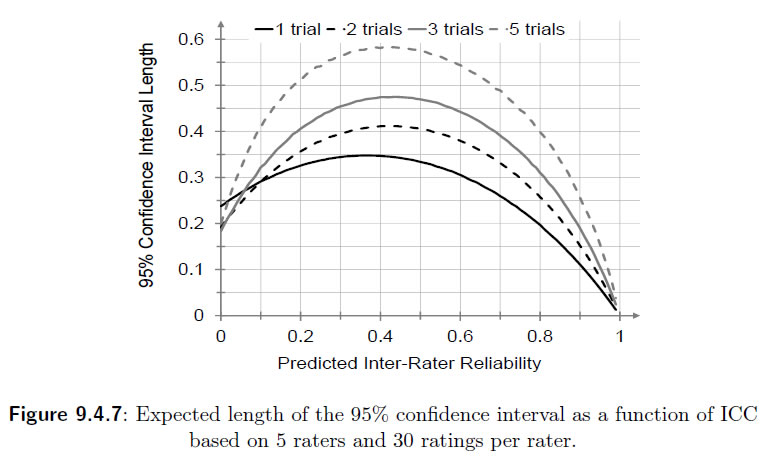Page 282 (last bullet point) "... the summation by M/n - r." must be replaced with "... the summation by M - rn." Pages 385-386 (Starting from "Examples" at the end of page 385) These examples contain a few errors.  The revised versions of these examples is the following: